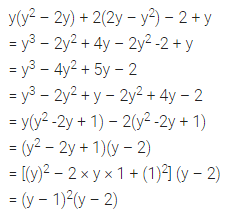# ML Aggarwal Class 8 Solutions for ICSE Maths Chapter 11 Factorisation Objective Type Questions

## ML Aggarwal Class 8 Solutions for ICSE Maths Chapter 11 Factorisation Objective Type Questions

Mental Maths
Question 1.
Fill in the blanks:
(i) When an algebraic expression can be written as the product of two or more expressions then each of these expressions is called ……….. of the given expression.
(ii) The process of finding two or more expressions whose product is the given expression is called ………..
(iii) HCF of two or more monomials = (HCF of their ……….. coefficients) × (HCF of their literal coefficients)
(iv) HCB of literal coefficients = product of each common literal raised to the ……….. power.
(v) To factorise the trinomial of the form x2 + px + q, we need to find two integers a and b such that a + b = ……….. and ab = ………..
(vi) To factorise the trinomial of the form ax2 + bx + c, where a, b and c are integers, we split b into two parts such that ……….. of these parts is b and there is ……….. ac.
Solution: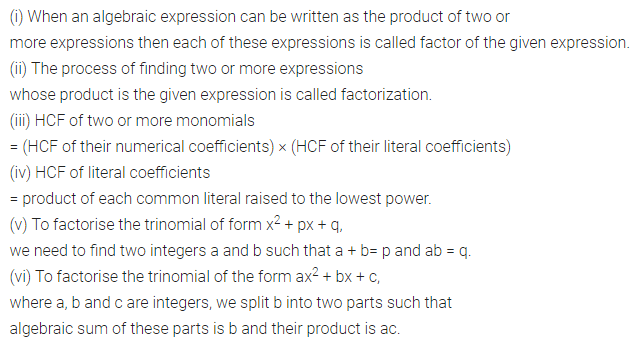Question 2.
State whether the following statements are true (T) or false (F):
(i) Factorisation is the reverse process of multiplication.
(ii) HCF of two or more polynomials (with integral coefficients) is the smallest common factor of the given polynomials.
(iii) HCF of 6x2y2 and 8xy3 is 2xy2.
(iv) Factorisation by grouping is possible only if the given polynomial contains an even number of terms.
(v) To factorise the trinomial of the form ax2 + bx + c where, a, b, c are integers we want to find two integers A and B such that
A + B = ac and AB = b
(vi) Factors of 4x2 – 12x + 9 are (2x – 3) (2x – 3).
Solution:Multiple Choice Questions
Choose the correct answer from the given four options (3 to 14):
Question 3.
H.C.F. of 6abc, 24ab2, 12a2b is
(a) 6ab
(b) 6ab2
(c) 6a2b
(d) 6abc
Solution: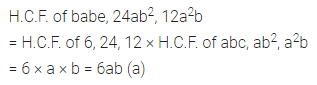Question 4.
Factors of 12a2b + 15ab2 are
(a) 3a(4ab + 5b2)
(b) 3ab(4a + 5b)
(c) 3b(4a2 + 5ab)
(d) none of these
Solution: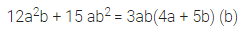Question 5.
Factors of 6xy – 4y + 6 – 9x are
(a) (3y – 2) (2x – 3)
(b) (3x – 2) (2y – 3)
(c) (2y – 3) (2 – 3x)
(d) none of these
Solution: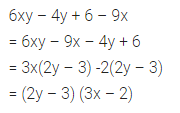Question 6.
Factors of 49p3q – 36pq are
(a) p(7p + 6q) (7p – 6q)
(b) q(7p – 6) (7p + 6)
(c) pq(7p + 6) (7p – 6)
(d) none of these
Solution: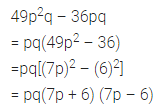Question 7.
Factors of y(y – z) + 9(z – y) are
(a) (y – z) (y + 9)
(b) (z – y) (y + 9)
(c) (y – z) (y – 9)
(d) none of these
Solution:Question 8.
Factors of (lm + l) + m + 1 are
(a) (lm + l )(m + l)
(b) (lm + m)(l + 1)
(c) l(m + 1)
(d) (l + 1)(m + 1)
Solution: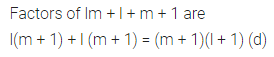Question 9.
Factors of z2 – 4z – 12 are
(a) (z + 6)(z – 2)
(b) (z – 6)(z + 2)
(c) (z – 6)(z – 2)
(d) (z + 6)(z + 2)
Solution:Question 10.
Factors of 63a2 – 112b2 are
(a) 63 (a – 2b)(a + 2b)
(b) 7(3a + 2b)(3a – 2b)
(c) 7(3a + 4b)(3a – 4b)
(d) none of these
Solution: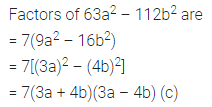Question 11.
Factors of p4 – 81 are
(a) (p2 – 9)(p2 + 9)
(b) (p + 3)2 (p – 3)2
(c) (p + 3) (p – 3) (p2 + 9)
(d) none of these
Solution:Question 12.
Factors of 3x + 7x – 6 are
(a) (3x – 2)(x + 3)
(b) (3x + 2) (x – 3)
(c) (3x – 2)(x – 3)
(d) (3x + 2) (x + 3)
Solution: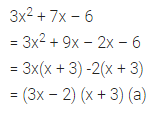Question 13.
Factors of 16x2 + 40x + 25 are
(a) (4x + 5)(4x + 5)
(b) (4x + 5)(4x – 5)
(c) (4x + 5)(4x + 8)
(d) none of these
Solution: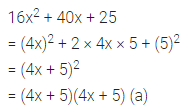Question 14.
Factors of x2 – 4xy + 4y2 are
(a) (x – 2y)(x + 2y)
(b) (x-2y)(x-2y)
(c) (x + 2y)(x + 2y)
(d) none of these
Solution: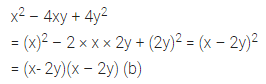Higher Order Thinking Skills (Hots)
Factorise the following
Question 1.
x2 + $$\left(a+\frac{1}{a}\right)$$x + 1
Solution:Question 2.
36a4 – 97a2b2 + 36b4
Solution: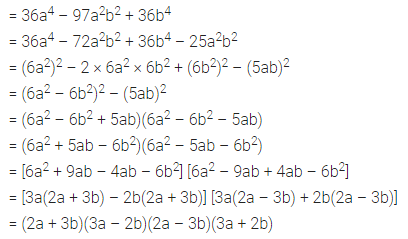Question 3.
2x2 – $$\sqrt{3}$$x – 3
Solution:Question 4.
y(y2 – 2y) + 2(2y – y2) – 2 + y
Solution: